# 1st PUC Maths Previous Year Question Paper March 2019 (South)

Students can Download 1st PUC Maths Previous Year Question Paper March 2019 (South), Karnataka 1st PUC Maths Model Question Papers with Answers helps you to revise the complete Karnataka State Board Syllabus and score more marks in your examinations.

## Karnataka 1st PUC Maths Previous Year Question Paper March 2019 (South)

Time: 3hrs 15 min
Max. Marks: 100

Instructions:

• The question paper has five parts A. B. C, D and E and answer all pails.
• Part-A carries 10 marks. Part-B carries 20 marks. Part-C carries 30 marks. part-D carries 20 marks, Pari-E carries 10 marks.

Part-A

Answer ALL the questions: (10 × 1 = 10)

Question 1.
Write the set (x : x ∈ R & -4 < x ≤ 6) as an interval.
(-4, 6).

Question 2.
Let A = {1, 2} and B= (3, 4). Find the number of relations from sets A to B.
No. of Relation = 2pq = 22 × 2 = 24 = 16 relations.

Question 3.
Convert $$\frac{7 \pi}{6}$$ into degree measure.
210°.Question 4.
Find the conjugate of $$\sqrt{3} i-1$$
– 1 – $$\sqrt{3} i$$

Question 5.
Find ‘n’ if nC7 = nC6
nCn-7 = nC6 ⇒ n – 7 = 6 ⇒ n = 13

Question 6.
Write the first three terms of the sequence an = (-1)n-1 5n+1
$$\frac{y^{2}}{4}-\frac{x^{2}}{9}=1$$
⇒ a2 = 4, b2 = 9
⇒ a = 4, b = 3
c = $$\sqrt{a^{2}+b^{2}}=\sqrt{4+9}=\sqrt{13}$$
The eccentricity e = $$\frac{c}{a}=\frac{\sqrt{13}}{2}$$

Question 7.
Find the slope of the line $$\frac{x}{3}+\frac{y}{2}=1$$
2x + 3y = 6, m = $$\frac{-a}{b}=\frac{-2}{3}$$

Question 8.
Evaluate $$\lim _{x \rightarrow 0} \frac{\cos x}{\pi-x}$$
$$\frac{\cos 0}{\pi-0}=\frac{1}{\pi}$$

Question 9.
Write the negative of “For every real number x, x is less than x + 1.”
There exist real number x, x is not less than x+1.

Question 10.
If $$\frac{2}{11}$$ is the probability of an event A, what is the probability of the event not A?
P(A’) = 1- P(A) = $$1-2 / 11=9 / 11$$

Part – B

Answer any TEN questions: (10 × 2 = 20 )

Question 11.
If U = {x : x ≤ 10, x ∈N} A = {x : x ∈ N and x is prime}, B = {x : x ∈ N. x is event} find A ∩ B im roaster form.
U = { 1, 2, 3, 4, …………….. 10} A = {2, 3, 5, 7}
B = {2, 4, 6, 8, 10}, A ∩ B = {2}

Question 12.
If x and y are the sets such that n(x) = 17, n(y) = 23 & n(X ∪ Y) = 38. Find n(X ∩ Y)
n(X ∩ Y) = n(X) + n(y) – n(X ∪ Y) = 17 + 23 – 38 = 2Question 13.
The cartesian product A × A has 9 elements among which are found (-1,0 & 0,1).
Find the set A and the remaining elements of A × A.
A = {-1,0, 1}
A × A = {(-1,-1),(-1,1), (0-1), (0,0),(1,-1), (1,1), (1,0)}

Question 14.
A wheel makes 360 revolutions in one minutes through how many radians does it turn in 1 second.
For one second, number of revolutions = 6.

Question 15.
If sin A = $$\frac{3}{5}$$ and A is in I quadrant then find sin2A..
sin A = $$\frac{3}{5}$$ cos A = $$\frac{4}{5}$$, sin 2A = 2sinAcosA = $$2\left(\frac{3}{5}\right)\left(\frac{4}{5}\right)$$ ⇒ sin 2A = $$\frac{24}{25}$$

Question 16.
Express $$i^{18}+\left(\frac{1}{i}\right)^{25}$$ in a + ib form.
$$i^{18}+\left(\frac{1}{i}\right)^{25}$$ = i2 + (-i)25 = i2 – i25 = i2 – i = -1 -i

Question 17.
Solve 3x – 2 < 2x + 1 & represent the solution graphically on the number line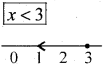Question 18.
Find the equation of the straight line intersecting y-axis at a distance of 2 units above the origin & making an angle 30° with the positive direction of x-axis.
c = 2, m = $$\frac{1}{\sqrt{3}}$$ ⇒ y = mx + c ⇒ y = $$\frac{1}{\sqrt{3}}$$ x + 2

Question 19.
Find the angle between the lines y – √3x – 5 = 0 and √3y – x + 6 = 0
y = $$\sqrt{3 x+5}$$ … (i) ∴ [m1 = √3]
√2y – x + 6 = 0 .
∴ √3y = x – 6 ∴ y = $$\frac{1}{\sqrt{3}} x-\frac{6}{\sqrt{3}}$$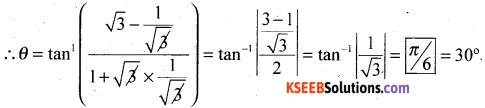Question 20.
Show that the points P(-2, 3, 5), Q (1,2,3) and R(7, 0, -1) are collinear.
A = (-2,3,5)
B = (1, 2, 3)
C = (7,0, -1)
By distance formula AB = √14, B = 2√14, CA = 3√14
∴ AC + BC = CA
∴ the points are collinear.

Question 21.
Evaluate $$\lim _{x \rightarrow 3} \frac{x-3}{x^{2}-5 x+6}$$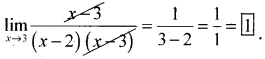Question 22.
Write the converse and contrapositive of the statement “If s is prime number then x is odd.”
Converse
If x is odd then x is primeContrapositive
If x is not odd then x is not prime.

Question 23.
The cofficient of vaiation and standard deviation are 60 and 21 respectively. What is the arithmetic mean of the distribution.
CV = 60 σ = 21 x̄ = ?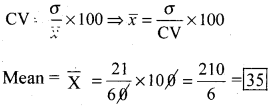Question 24.
Given p(A) = $$\frac { 3 }{ 5 }$$ and p(B) = $$\frac { 1 }{ 5 }$$. Find P(A or B), if A & B are mutually exclusive events.
P(A ∪ B) = p(A) + p(B) (∵p(A ∩ B) = 0)
$$=\frac{3}{5}+\frac{1}{5}=\left[\frac{4}{5}\right]$$

Part – C

Answer any TEN questions: (10 × 3 = 30)

Question 25.
In a group of 600 students in a school, 150 students were found to be taking tea, 225 and taking coffee and 100 were taking both tea and coffee. How may students were taking neither tea nor coffee.
Let set of students taking coffee = C & set of students taking tea = T
Also set of students who take either Tea or Coffee = C ∪ T
n (T) = 150 n(C) = 225
n(C ∩ T) = 100 ; n(C ∪ T) = ?
n(C ∪ T) = n(C) + n(T) – n (C ∩ T)
= 150 + 225 – 100 = 275
Number of students taking neither Tea nor Coffee
= n(∪), n (C ∪ T) = 600 – 275 = 325

Question 26.
Let A = {1. 2. 3……… 141. Define a relation R from A to A by R = {(x, y): 3x – y = 0 x,y ε A}. Write ‘R’ in roaster form, write its Domain and Range.
y = 3 x R = {(1,3),(2,6), (3,9), (4, 12)}
Domain of R = {1, 2, 3, 4}
Range of R = {3, 6, 9, 12)
Codomain of R = {1, 2, 3, ….. 14}

Question 27.
Find the general solution of 2cos2x + 3sinx = 0.
2cos22x + 3sinx = 0
2(1 – sin22x) + 3sinx = 0
2 – 2sin22x + 3sinx = 0
2sin22x – 3sinx – 2 = 0
2sin22x – 4sinx + sinx – 2 = 0
2sin x(sin x – 2)+1 (sinx – 2) = 0
(sin x – 2)(2sin x + 1) = 0
sin x – 2 = 0 or 2 sin x + 1 = 0
sin x = 2 or sin x = -1/2

Question 28.
Express $$\frac{-1+i}{\sqrt{2}}$$ in the polar form.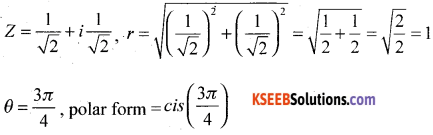Question 29.
Solve the equation $$x^{2}+\frac{x}{\sqrt{2}}+1=0$$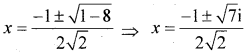Question 30.
How many words with or without meaning can be made from the letters of the word ‘MONDAY’ assuming no letter is repeated. if
(i) 4 letters are used at a time.
(ii) All letters are used at a time
(iii) All letters are used but first letter is a vowel.
There are 6 letters in the word MONDAY. So, the total number of words is equal to the number of arrangements of these letters taken four at a time
The number of such arrangements = 6P4 $$=\frac{6 !}{(6-4) !}=\frac{6 !}{2 !}=\frac{6.5 .4 .3 .2 .1}{2.1}=360$$
Hence, total number of words = 360
(ii) Total number of arrangements in this case = 6P6 = 6! = 720
(iii) Total number of arrangements when all letters are used but the first letter is a vowel
= 2. 5P5 = 2.5! = 2 × 5 × 4 × 3 × 2 x 1 = 240.

Question 31.
Find the term independent of x in the expansion of $$\left(\frac{3 x^{2}}{2}-\frac{1}{3 x}\right)^{6}$$
We have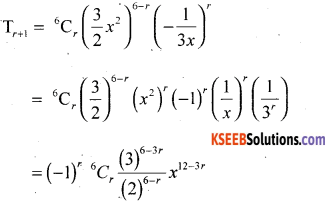The term will be independent of x if the index of x is zero, i.e., 12 – 3r = 0. Thus, r= 4
Hence 5th term is independent of x and is given by (-1)4 6C4 $$\frac{(3)^{6-8}}{(2)^{6-4}}=\left[\frac{5}{12}\right]$$

Question 32.
The sum of first three terms of a GP is $$\frac{13}{12}$$ and their product is –1. Find the common ratio and the terms.
Given Let the numbers be $$\frac{\mathrm{a}}{\mathrm{r}}$$, a & ar.
Product = -1
∴ $$\frac{\mathrm{a}}{\mathrm{r}}$$.a.ar = -1
a3 = -1 ⇒ a = -1Given Sum = $$\left[\frac{13}{12}\right]$$– 12 – 12r2 = 25r
12r2 + 25r + 12 = 0
12r2 + 16 + 9r + 12 = 0.
4r(3r + 4) + 9r + 12.= 0
4r(3r + r) + 3(3r + 4) = 0
(3r + 4)(4r + 3) = 0
$$r=-\frac{4}{3},-\frac{3}{4}$$
∴ the numbers are
(i) $$\frac{a}{r}=\frac{-1}{-4 / 3}=\frac{3}{4}$$
(ii) a = -1
(iii) ar = -1. $$\left(-\frac{4}{3}\right)=\frac{3}{4}$$
$$\frac{3}{4},-1, \frac{4}{3}$$Question 33.
Insert 3 arithmetic means between 8 & 24.
an = a + (n + 1) d
24 = 8 + (y – 1)d ∴ 16 = 4d ⇒ d=4
3 Means are [12,16,20 ]

Question 34.
Find the equation of the parabola with vertex at the origin, axis along x – axis and passing through the point (2, 3) also find its focus,
y2 = 4ax, (2,3) ⇒ 9 = 4a(2) ⇒ a = $$\frac{9}{8}$$
Equn. is y2 = 4$$\left(\frac{9}{8}\right)$$ x ⇒ y2 = $$\frac{9}{2} x$$ Focus = $$\left(\frac{9}{8}, 0\right)$$

Question 35.
Find the derivative of the function cosx w.r. t. x from first principle.
Let f(x) = cos x. ∴ f(x + ∆x) = cos(x + ∆x).Question 36.
Verify by the method of contradiction that √2 is an irrational,
If possible Let √2 -be rational
∴ √2 = $$\frac { p }{ q }$$ where p, q ∈z, q ≠ 0 we also assume that p and q do not have any common factor
∴ √2q = p ⇒ 2q2 = p2 ⇒ p2 is even
⇒ p is even

Put
∴ p = 2k where k ∈ z
p2 = 4k2
2q2 = 4k2 ⇒ q2 = 2k2 ⇒ q2 is even
⇒ q is even

∴ p and q are both even and hence has a common factor 2 which is a contradiction
∴ √2 is irrational.Question 37.
One card is drawn from a well-shuffled deck of 52 cards. If each out come is equally likely, calculate the probability that the card will be (i) diamond (ii) not an ace (iii) a black card,
(i) Let A: Card drawn is diamond.
∴ n(A) = 13 → favourable number of events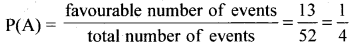(ii) P(Ā) = P(not A) = 1 – P(A) = $$1-\frac{1}{4}=\frac{3}{4}$$

(iii) There are 26 black cards
∴ p(black) = $$\frac{26}{52}=\frac{1}{2}$$

Question 38.
A fair coin 1 marked on one face and 6 on the other face and a fair die are both tossed. find the probability that the sum of numbers that turn up is (i) 3 (ii) 12.
Coin has faces marked with 1, 6.
A fair die has faces marked as 1, 2, 3, 4, 5, 6.
∴ total number of equally likely cases = 2 × 6 = 12
(i) Favourable case is (1, 2) i.e., 1 only. ∴ red. probability = $$\frac { 1 }{ 12 }$$
(ii) Favourable case is (6, 6) i.e. 1 only. ∴ reqd. probability = $$\frac { 1 }{ 12 }$$

Part -D

Answer any SIX questions: (6 × 5 = 30 )

Question 39.
Define signum function. Draw the graph of it and Write down its domain and Range.
The function f: R → R defined byis called the signum function. The domain of the signum function is R and the range is the set {-1,0, 1}.Question 40.
Prove that $$\frac{\cos 4 x+\cos 3 x+\cos 2 x}{\sin 4 x+\sin 3 x+\sin 2 x}=\cot 3 x$$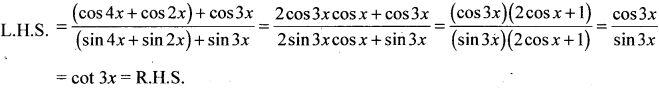Question 41.
Prove by mathematical induction
12 + 22 + 32 + …… + n2 = $$\frac{n(n+1)(2 n+1)}{6}$$ ∀n ∈ N
Let p(n) : 12 + 22 + 32 + …… + n2 = $$\frac{n(n+1)(2 n+1)}{6}$$
step 1 : Prove that P(1) is true
when n= 1; L.H.S = 12 = 1 R.H.S = 1(1 + 1)(2 + 1)6 = 2.3/6 = 1
∴ L.H.S = R.H.S
∴ P(1) is true

step 2: Assume that P(m) is true
i.e., 12 + 22 + 32 + ………. + m2 = $$\frac{n(m+1)(2 m+1)}{6}$$ ……… (1)

step 3: Prove that P(m + 1) is true
i.e., 12 + 22 + 32 + ……….. m2 + (m+1)2 = $$\frac{(m+1)(m+2)(2 m+3)}{6}$$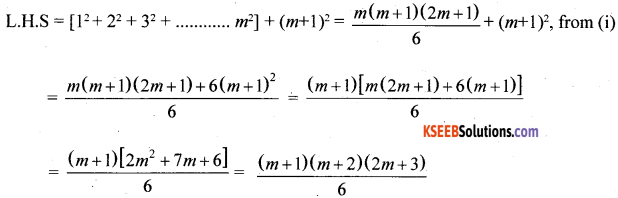Conclusion: P(1) is true
P(m) is true ⇒ P(m + 1) is true
∴ By principle of mathematical induction, the result is true for all natural numbers ‘n’.

Question 42.
Solve graohically the system of inequalities x + 2y ≥ 20, 3x + y ≤ 15
Consider the equations
x + 2y = 20 … (1) and 3x + y = 15 ….(2)(i). Region represented by x + 2y ≥ 20 :
line (1) meets x-axis it (20,0) and y-axis at (0, 10) By joining these two points we get the line (1) put x = 0, y = 0 in x + 2y ≥ 20 ⇒ 0 ≥ 20, which is not true
∴ (0,0) does not satisfy the inequation
∴ The portion which does not contain origin represents the solution set of x + 2y ≥ 20

(ii) Region represented by 3x + y ≤ 15
line (2) meets x-axis at (5,0) and y-axis at (0, 15) By joining these two, we get line (2)
∴ put x = 0, y = 0 in 3x + y ≤ 15 ⇒ 0 ≤ 15 which is true
∴ (0, 0) satisfies the inequation
∴ The portion containing the origin represent the solution set of 3x + y = 15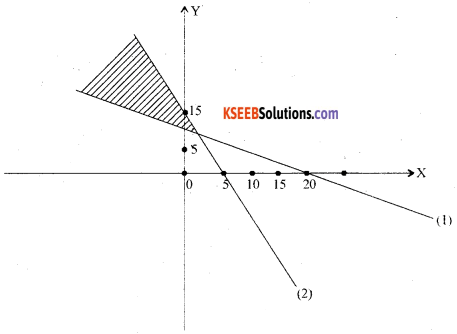∴ All the points in the common shaded region are the solutions of the system of linear in equationsQuestion 43.
A group consists of 4 girls and 7 boys. In how many ways can a team of 5 members be en selected, if the team has at “least one boy and one girl”?
(i) Since, the team will not include any girl, therefore, only boys are to be selected. 5 boys out of 7 boys can be selected in 7C5 ways. Therefore, the required number of ways
$$=^{7} \mathrm{C}_{5} \frac{7 !}{5 ! 2 !}=\frac{6 \times 7}{2}=21$$

(ii) Since, at least one boy and one girl are to be there in every team. Therefore, the team can
consist of
(a) 1 boy and 4 girls
(b) 2 boys and 3 girls
(c) 3 boys and 2 girls
(d) 4 boys and 1 girl.
1 boys and 4 girls can be selected in 7C1 × 4C4 ways.
2 boys and 3 girls can be selected in 7C2 × 4C3 ways.
3 boys and 2 girls can be selected in 7C3 × 4C2 ways.
4 boys and 1 girls can be selected in 7C4 × 4C1 ways.
Therefore, the required number of ways
= 7C1 × 4C4 + 7C2 × 4C3 + 7C × 4C2 + 7C4 × 4C1
= 7 + 84 + 210 + 140 = 441

(iii) Since, the team has to consist of at least 3 girls, the team can consist of
(a) 3 girls and 2 boys, or (b) 4 girls and 1 boy.
Note that the team cannot have all 5 girls, because, the group has only 4 girls.
3 girls and 2 boys can be selected in 4C3 × 7C2 ways.
4 girls and 1 boys can be selected in 4C4 × 7C2 ways.
Therefore, the required number of ways
= 4C3 × 7C2 + 4C4 × 7C1 = 84 + 7 =91.

Question 44.
State and prove Binomial theorem for positive integral index ‘n’.
Statement: “If ‘n’ is a +ve integer then
(x + a)n = nc0 xn a0 + nc1 xn-1 a1+ n2 xn-2.a2+ ………….. + ncn x0 an.
Proof : (By Mathematical induction):
Let p(n) : (x + a)n = nc0 xna0 + nc1 xn-1 a1 + nC2 xn-2 a2 + ……………… + ncn x0an.

Step1 : Prove that P(1) is true
when n= 1, L.H.S = (x + a)1 = x + a; RHS = 1c0 x1 a1 = |x.| + 1.1.a = x + a
L.H.S. = R.H.S.
∴ P(1) is true

Question 45.
Find the co- ordinate of the foot of the perpendicular from the point (-1,3) to the line 3x – 4y – 16 = 0.
Let (a, b) be the coordinates of the foot of perpendicular from the point (-1,3) to the line and
slope of the line joining (-1,3) and (a, b) = $$\frac{b-3}{a+1}$$
Slope of the line 3x – 4y – 16 = 0 is = $$\frac{3}{4}$$
Since two lines are perpendicular $$\left(\frac{b-3}{a+1}\right)\left(\frac{3}{4}\right)=-1$$
4a + 3b = 5 ………….. (1)
Point (a b) lies on 3x – 4y – 16 = 0
3a – 4b – 16 = 0 …………. (2)
Solving (1) & (2), we get a = $$\frac{68}{25}$$  b = $$\frac{49}{25}$$Question 46.
Derive an expression for the coordinates of a point that divides the line joining the points A(x, y, z,) & B(x2 y2 z2) internally in the ratio m: n and hence find the mid-point of(1,2,3) & (5,6,7)
Section formula
Obtain the co-ordinates of the point which divides the line joining A(x1 y1, z1,) and B(x2, y2, z2)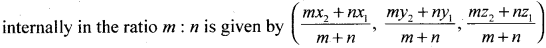Proof: Let P(x1, y1, z1,) and Q(x2, y2, z2) be the given points. Let R(x, y, z) divide PQ internally in the ratio m:n.
Draw PL, QM, RN ⊥r‘ to XY-plane.”
∴ PL ∥ RN ∥ QM
∴ PL, QM, RN lie in one plane so that the points L, N, M lie in a straight line which is the intersection of this plane and XY plane, through the point R draw a line AB parallel to the line LM. The line AB will intersect the line LP externally at A and the line MQ at B.
Triangles APR and BQR are similar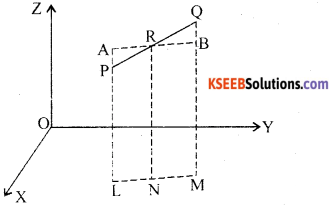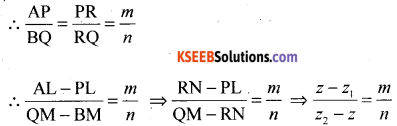∴ n(z – z1) = m(z2 – z) ⇒ nz – nz1 = mz2 – mz
mż + nz = mz2 + nz1 ⇒ (m + n)z = mz2 + nz1 ⇒ z = $$\frac{m z_{2}+n z_{1}}{m+n}$$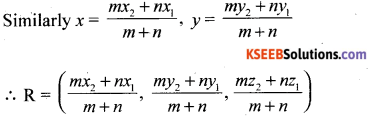Question 47.
Prove that $$\lim _{\theta \rightarrow 0} \frac{\sin \theta}{\theta}=1$$ (θ is measured in radian) and hence evaluate $$\lim _{\theta \rightarrow 0} \frac{\sin a \theta}{\sin b \theta}$$
Proof :
Consider a circle with centre O & radius ‘r’ Mark two points A & B on the circle so thatAt A draw a tangent to the circle. Produce OB to cut the tangent at C.
Join AB. Draw BM ⊥ OA
From the figure it is clear that
Area of ∆OAB < Area of sector OAB < sector of ∆OAC ….. (1)
Area of ∆OAB = $$\frac { 1 }{ 2 }$$ OA.BM
[In ∆ OBM.Sinθ = $$\frac{B M}{O B}=\frac{B M}{r}$$ ⇒ BM = r Sinθ]∴ Area of ∆OAB = $$\frac{1}{2} r$$ r.sinθ = $$\frac{1}{2} r^{2}$$ sinθ
Area of sector OAB = $$\frac{1}{2} r^{2} \theta$$
Area of ∆OAC = $$\frac{1}{2} \mathrm{OA} \cdot \mathrm{AC}$$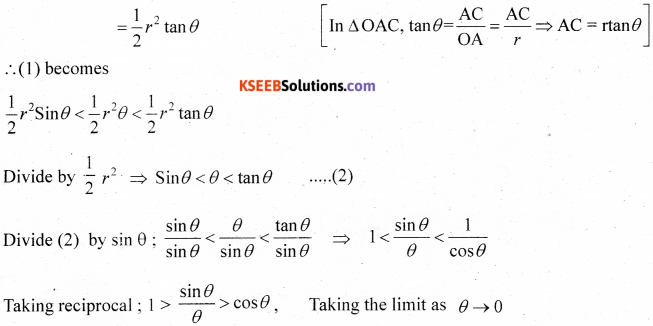Question 48.
Find the mean deviation about mean for the following data.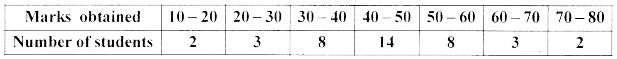0 = 10Part – E

Answer any ONE question: (1 × 10 = 10)

Question 49.
(a) Prove geometrically that cos (x + y) = cos x cosy – sinx siny.
Prove that cos(x + y) = cos x cos y .sin x sin yProot Consider a unit circle (radius = 1 unit) with centre is (0, 0).
Consider 4 point and P1, p2, p3 and p4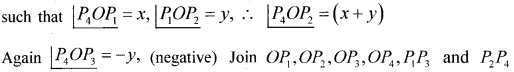The co-ordinate of and P1, p2, p3 and p4 are given by
p1 = (cosx,sinx) P2 = [cos(x+y), sin(x+y)]
p3 = [cos(-y),sin (-y)] P4 = [1,O]
From the figure OP1OP3 is congruent to P2P4
∴ From distance formula
p1p3 = p2p4 …(l)
Take the distance (p1P3 )2 = [cosx – cos(-y)]2 + [sin x – sin (-y)]2
= (cosx – cosy)2 + (sin x + sin y)2
= cos2 x + cos2 y = cosxcosy + sin2x + sin2y + 2sincosy = 1 + 1 + 2(cosxcosy – sinxsiny)
(P1P3)2 = 2 – 2 cos(x + y)
Again (P2P4 )2 = [1(a-b)2-cos(x+y) ]2 + |q – sin (x + y)|2
= 1 + cos2(x + y) -2cos(x + y) + sin2(x + y) = 1 + 1 – 2cos(x + y) = 2 – 2cos(x + y)⇒ LHS = RHS
∴ [cos(x + y) = cosxcosy – sin x sin y]

(ii) Show that cos2x = cos2x – sin x2x
Take cos(x + y) = cosxcos y – sin xsin y
Put y = X
∴ cos(x + x) cosxcosx – sin xsin x
cos2x = cos2x – sin2x(b) Find the derivative of $$\frac{x^{5}-\cos x}{\sin x}$$ w.r.t. x.Question 50.
(a) Define ellipse and derive its equation in the form $$\frac{x^{2}}{a^{2}}+\frac{y^{2}}{b^{2}}$$ =1(a > b)
Let F1 and F2 be the foci and O be the mid point of the line segment F1F2. Let O be the origin
and the line from 0 through F2 be the positive x-axis and that through F1 as the negative x – axis. Let, the line through O perpendicular to the x-axis be the y-axis. Let the coordinates of F1 be (-0, 0) and F2 be (c, 0).
Let P(x, y) be any point on the ellipse such that the sum of the distances from P to the two foci be 2a .
i.e., PF1 + PF2 = 2a. ….(1)
Using the distance formula, we have
$$\sqrt{(x+c)^{2}+y^{2}}+\sqrt{(x-c)^{2}+y^{2}}=2 a$$
$$\text { ie., } \sqrt{(x+c)^{2}+y^{2}}=2 a-\sqrt{(x-c)^{2}+y^{2}}$$
Squaring both sides, we get.
(x + c)2 + y2 = 4a2 – 4a $$\sqrt{(x-c)^{2}+y^{2}}+(x-c)^{2}+y^{2}$$
which on simplification gives
$$\sqrt{(x-c)^{2}+y^{2}}=a-\frac{c}{a} x$$
Squaring again and simplifying, we get
$$\frac{x^{2}}{a^{2}}+\frac{y^{2}}{a^{2}-c^{2}}=1$$
i.e., $$\frac{x^{2}}{a^{2}}+\frac{y^{2}}{b^{2}}=1$$ (since c2 = a2 – b2)
Hence any point on the ellipse satisfies
$$\frac{x^{2}}{a^{2}}+\frac{y^{2}}{b^{2}}=1$$
Conversely, let P(x, y) satisfy the equation (2) with 0 < c < a. ThenSo, any point that satisfies $$\frac{x^{2}}{a^{2}}+\frac{y^{2}}{b^{2}}=1$$ satisfies the geometric condition and so P(x, y) lies
on the ellipse.

Hence from (2) and (3), we proved that the equation of an ellipse with centre of the origin and major axis along the x-axis is
$$\frac{x^{2}}{a^{2}}+\frac{y^{2}}{b^{2}}=1$$(b) Find the sum to n terms of the series 3 × 8 + 6 × 11 + 9 × 14 + …………….. 4